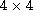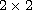Electron. J. Diff. Equ., Vol. 2015 (2015), No. 212, pp. 1-16.

### A matrix formulation of Frobenius power series solutions using products ofmatrices Jeremy Mandelkern

Abstract:
In Coddington and Levison [7, p. 119, Thm. 4.1] and Balser [4, p. 18-19, Thm. 5], matrix formulations of Frobenius theory, near a regular singular point, are given usingmatrix recurrence relations yielding fundamental matrices consisting of two linearly independent solutions together with their quasi-derivatives. In this article we apply a reformulation of these matrix methods to the Bessel equation of nonintegral order. The reformulated approach of this article differs from  and  by its implementation of a new vectorization'' procedure that yields recurrence relations of an altogether different form: namely, it replaces the implicitmatrix recurrence relations of both  and  by explicitmatrix recurrence relations that are implemented by means only ofmatrix products. This new idea of using a vectorization procedure may further enable the development of symbolic manipulator programs for matrix forms of the Frobenius theory.

Submitted January 12, 2015. Published August 17, 2015.
Math Subject Classifications: 34B30, 33C10, 68W30, 34-03, 01A55.
Key Words: Matrix power series; Frobenius theory; Bessel equation.

An addendum was posted on May 11, 2017, It modifies two matrices from Section 7. See the last page of this article.

Show me the PDF file (284 KB), TEX file, and other files for this article.Jeremy Mandelkern Department of Mathematics Eastern Florida State College Melbourne, FL 32935, USA email: mandelkernj@easternflorida.edu